# Left Function in Microsoft Excel

In this article, we’ll learn how we can use LEFT function in Microsoft Excel.

In Microsoft Excel, LEFT function is used to extract the leftmost characters from a string. In addition, the number of characters returned from the left side is based on the number of characters we specify. It is generally used to separate text, like initial, first name, zip code, area code etc.

LEFT function takes two arguments:

First is “text”, it’s the text / string from which we want to extract characters.

And second is “[num_char]”, it is an optional argument and if omitted, it is assumed to be 1.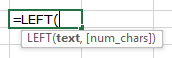Let’s take examples and understand,

We have taken sample data according to usage of LEFT function in different situations.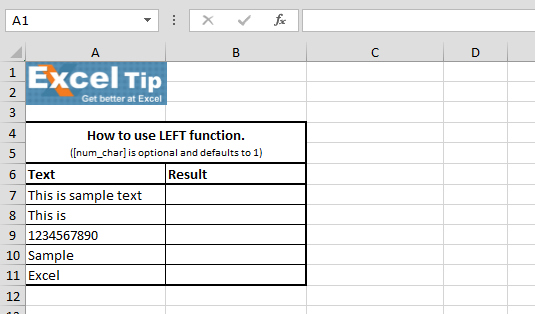1st Example:-

In the first example, we will learn how we can extract 4 letters from left.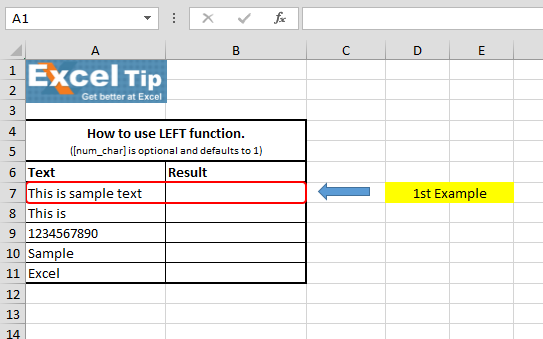• Enter the function in cell B7
• =LEFT(A7,4)
• Press Enter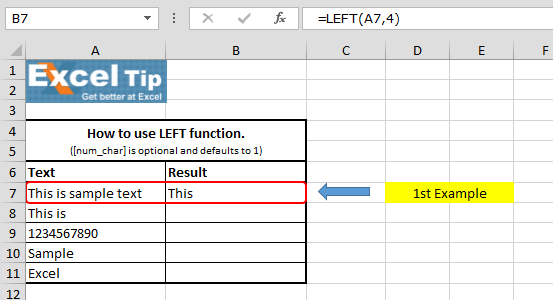It returns the left 4 characters which is “This” from the text “This is simple text”.

2nd Example:-

And, what happens if we do not enter anything in the 2nd argument and skip it?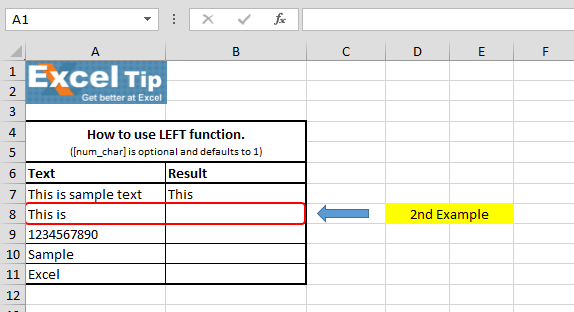• Enter the function in cell B8
• =LEFT(A8), Press Enter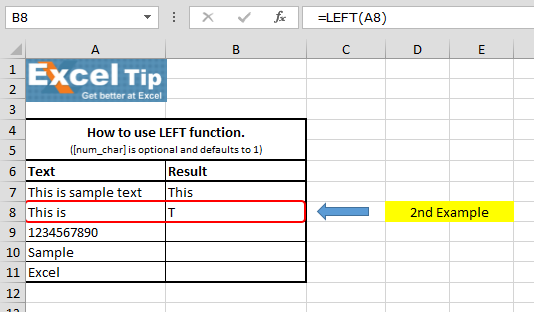It gives us “T” because by default, it has extracted the first left character from the string.

3rd Example:-

In the below image, we want to extract “12345” in cell B9 from the longer number “1234567890” located in cell A9.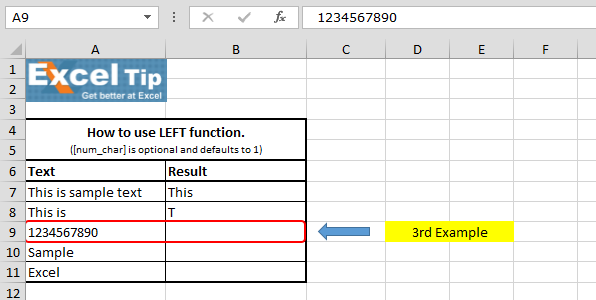• Enter the function in cell B9
• =LEFT(A9,5)
• Press Enter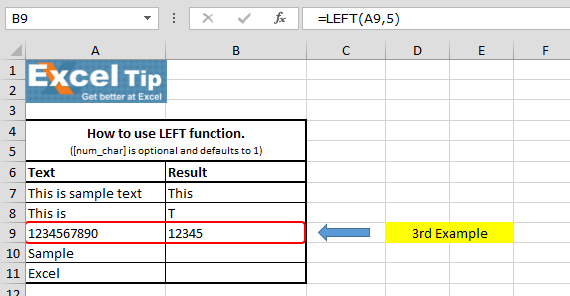4th Example:-

In this example, we’ll see that if we give 0 in the 2nd argument then what will be the result.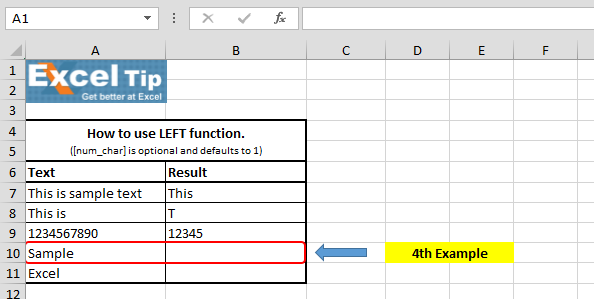• Enter the function in cell B10
• =LEFT(A10,0), Press Enter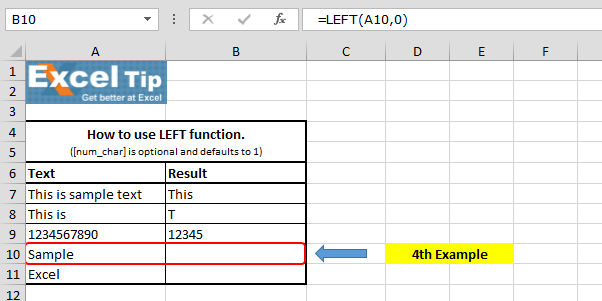The function has returned empty cell because we have specified 0 in number of characters we wanted to extract from the string.

5th Example:-

In this example, we will enter negative number in the second argument.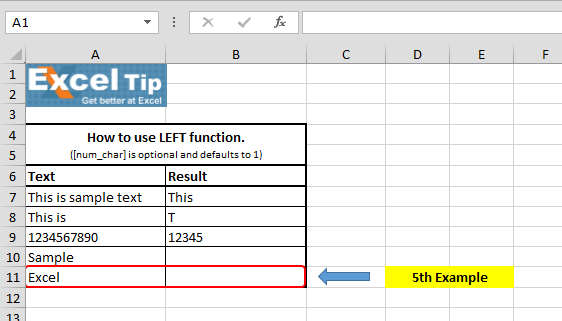• Enter the function in cell B11
• =LEFT(A11,-1), Press Enter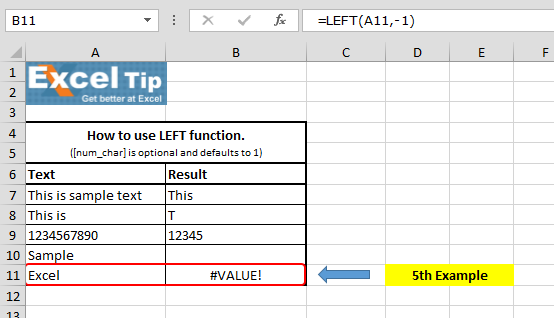It gives #VALUE error because the num_char is less than 0, and LEFT does not allow the negative value.

So, this is how we can use LEFT function in different situations.

# Video: How to use LEFT function in Microsoft Excel

Watch the steps in this short video, and the written instructions are above the video